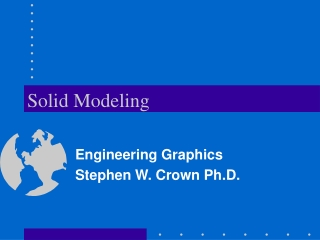DownloadDownload PresentationSolid Modeling

# Solid Modeling

Download Presentation## Solid Modeling

- - - - - - - - - - - - - - - - - - - - - - - - - - - E N D - - - - - - - - - - - - - - - - - - - - - - - - - - -
##### Presentation Transcript

1. Solid Modeling Engineering Graphics Stephen W. Crown Ph.D.

2. Objective • To learn how to generate solid models in CAD • To understand how to use solid modeling in the design process

3. Overview • Primitives • Extrude • Sweep • Revolve • Blend • Solid Model Operators • Viewing Solid Models

4. Solid Models • Definition: A 3-D model of an object that contains volumetric information • Mass can be represented • Interior surfaces are generated automatically as portions of an object are removed • Easily constructed using primitive shapes, extruding, and revolving

5. Solid Primitives • Box (Parallelepiped) • Cylinder • Cone • Sphere • Wedge

6. Example: Using Primitives • Box • Wedge • Union / Protrusion • 6 boxes • 2 wedges • Subtract / Cut • 1 box - minus 2 wedges • 1 box - minus 2 boxes

7. EXTRUDE • Two dimensional closed objects can be extruded to give solid objects • The direction of the extrusion is typically normal to the 2-D cross sectional sketch • The height of extrusion can be specified

8. SWEEP • The path of the extrusion must be defined (trajectory) • The extruded cross section must be defined • The cross section stays normal to the path

9. REVOLVE • Two dimensional closed objects can be revolved to give solid objects • The axis of rotation must be defined • The angle of revolution must be specified

10. BLEND • Smooth transition can be made between two closed shapes with similar geometry (i.e. equal number of vertices) • The distance between sections must be defined • The angle of twist between sections must be specified

11. Solid Model Operators • Subtract / Cut • Intersection • Union / Protrusion

12. Solid Model Operators • Subtract / Cut • subtracts one solid from another • One solid is subtracted from the other.

13. Solid Model Operators • Intersection • Creates a solid that represents the region that is in common to the selected solids

14. Solid Model Operators • Union / Protrusion • Creates a single solid from two solids that intersect

15. Example: Solid Model Operators What procedure would you follow to create the two mating parts? • Create the bounding box for the upper part • Create the two cylinders • Subtract the cylinders from the box • Create the bounding box for the lower part • Subtract the finished upper part

16. Example: Solids from Drawings • Extrude the view with the most detailed perimeter • The depth of the extrusion may be found from an adjacent view

17. Example: Solids from Drawings • Use the perimeter of other views to make additional cuts • Interpret interior details by making assumptions about geometry in one view and testing them in adjacent views

18. Example: Solids from Drawings • Verify that the completed part satisfies every view • Generate a multiview drawing from the solid part and compare to the original sketch or drawing

19. Using Solid Modeling for Design • Intersection of wedge and cylinders is not clearly defined • Construction of solid model forces resolution regarding details of construction

20. Using Solid Modeling for Design • Intersection of wedge with post produces a gap, a ledge, or a circular groove • Intersection of wedge with base produces a ledge, an overhang, or requires a rounded end • These details can be shown in the multiview drawing but are more obvious in the solid model

21. Viewing Solid Models • Rendering Styles • Wireframe with hidden lines • Hidden lines removed • Shaded

22. Viewing Solid Models • LIGHTING • Distance, Point, Spotlight • Ambient light • Intensity • Modify (specify location of light) • SURFACE FINISH • Specify roughness, color, sensitivity to ambient light, texture maps, and bump maps • Apply finishes to individual parts or assemblies